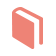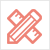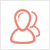﻿ 更多范文-如何通过深度来加深记忆--Assignment代写范文-51Due留学教育

#### 服务承诺资金托管原创保证实力保障24小时客服使命必达

#### 关于我们

51Due提供Essay，Paper，Report，Assignment等学科作业的代写与辅导，同时涵盖Personal Statement，转学申请等留学文书代写。51Due将让你达成学业目标51Due将让你达成学业目标51Due将让你达成学业目标51Due将让你达成学业目标

#### 名企实习积累工作经验多元化文化交流专业实操技能建立人际资源圈

# 如何通过深度来加深记忆--Assignment代写范文

2017-03-04 来源: 51Due教员组 类别: 更多范文

Assignment代写范文:“如何通过深度来加深记忆”，这篇论文主要描述的是记忆是我们大脑对于事或物的回放和重现，这也是一种人类特有的高级心理活动现象，我们对于人类记忆的研究与学习，能够让我们以科学的方式更好的去改善人类现有的记忆学习能力，人在一定时间能够执行和处理的信息量也就是我们所说的记忆容量，我们所保存的大部分记忆都是以短期记忆的形式，在一个短时间后被我们所遗忘。Abstract 摘要

Memory is a common topic in the process of the research. In addition, in the research, the author would like to apply the paired sample t-test to adjust the processing of the memory. In this research, the three key values are fully applied: t-value, the degree of the freedom and p-value, so that these three values could defined the finally to prove the relationship for the memories.

1.0 Introduction 简介

Memory is the recognition of the human mind to remember things, keeping, reproduce or recognize. It is to carry out thinking, imagination and other high-level psychological activities. Human memory and brain structure of the hippocampus, the brain changes in the chemical composition.Memory as a basic psychological process, and other psychological activities are closely linked. Memory links with people's mental activities (Dutriaux&Gyselinck, 2016). Memory is the basic function of people learning, working and living. The process of abstract disorder into image order is the key of memory.The study of memory belongs to the category of psychology or brain science. Modern human memory research continues, although today's science and technology has been developed by leaps and bounds. The use of those after practice can effectively improve the memory of the methods, skills, can make it better serve the human work, dailylife, and learning (von Stülpnagel, Steffens, &Schult, 2016).

While learning is seen as an obvious part of schooling, this makes it a process of concealment and teacher observation, re-instruction, or immediate corrective inaccessibility. An important aspect of learning that is often taken for granted is that learners are successfully involved in multiple messages simultaneously, following multiple step directions, solving problems, or through a lesson or teaching objectives such as self-managing implicit needs such as complex thinking, e.g., tracking of the accumulation over an extended period of time (Moreira, et al., 2015). However, this seemingly basic ability is complex and involves well-coordinated cognitive processes between at least three administrative functions: inhibitory control, working memory renewal, and mental transfer The typical characteristic of working memory capacity is that the information individual can Processing the same time to perform complex tasks.

Normally working memory capacity is characterized as being within the range of information that the individual can process at the same time to perform complex tasks. The larger a person's capacities, the more powerful the attention can be controlled to effectively manipulate the information and avoid processing disturbances. This psychological multitasking is achieved by the parallel processing of short-term memory from a person's attention from the highly activated long-term memory access or temporal information coordination and timely control appearing (Antoniou, Ettlinger, & Wong, 2016). In this way, it can be processed according to need, like a mental spotlight selectively according to the relevant information from one moment to another working memory function, actively do the relevant material original intention. The ineffective operation of this working memory spotlight increases the risk that distracting information will be allowed to dispose of irrelevant information, the ability to overload this information is limited, and obstructing effective spotlighting can lead to forgotten ways of shifting the mindset.

2.0 Method 方法

2.1 Design

In the process of the research of the processing of the memory, the paired sample t-test method is applied. In addition, the definition of the paired sample t-test can be summarized as follow:

The paired sample t-test was used to compare the two demographic techniques in the case of the relevant two samples, the device. The paired sample t test is used in the 'anterior - posterior' study, or when the sample was matched, or when it was a case - control study. In addition, the paired t test is similar to the repeated measurement ANOVA test. In addition, it can be used to compare the performance of static groups under different test conditions. The difference is that paired t-tests are used when the independent variable has two levels. Repeat measures ANOVA when independent variables have more than two levels used. Thus, for example, if a researcher tests three conditions, the appropriate test will be repeated for ANOVA, not a paired t-test. In addition, values are reported: t price, degree of freedom and P value (NikoueiMahani, 2016).

2.2 Participants

There are three hundred and seventy-nine participantstaking part in the study. They are supposed cover genders, ages, races and all other personal marks as much as possible. Though due to the location of this questionnaire is taken place, and the number of participants is comparatively low, the participants can be only comparatively scientific.

2.3 Materials

The random sample would like to link to memory students and also refer to the academic journals to reference to the study.

2.4 Procedures

In the process of the research, the paired samples t-test is used to the comparing deep VS shallow levels of processing.Three hundred and seventy-nine participants are randomly selected. They are supposed cover genders, ages, races and all other personal marks as much as possible. In this process, they are given to a paper of list of questions. In order to avoid environmental influences and personal and subjective bias, the participants are required to finish the questions independently.When the questions are finished, the paper is collected immediately.

3.0 Results 结果

Paired Samples statistics

Mean    N    Std. Deviation    Std. Error Mean

Pair 1 Pleasure/Unpleased    10.7968    379    2.6802    0.13767

E or not    8.1821    379    2.89427    0.14867

Figure 1: the paired samples statistics for the processing of the memory

Paired Samples Correlations

N    Correlation    Sig.

Pair 1 Pleasant/Unpleasant & E or not    379    0.392    0

Figure 2:  the paired samples correlation for the processing of the memory

Paired Samples Test

Paired Differences

Mean    Std. Deviation    Std. Error Mean    95% Confidence Internal of the Difference    　    t    df    Sig (2-tailed)

Lower    Upper

Pair 1 Pleasant/Unpleasant - E or not    2.61478    3.0791    0.15816    2.30379    2.92576    16.532    378    0

Figure 3: the paired samples test for the processing of the memory

As can be seen in the Figure 1, the mean is 10.8 and standard deviation is 7.68 for the category of the “pleasant” and also mean is 8.18 and standard deviation is 2.89 for the category of “unpleased”.

Besides that, the Figure 3 has demonstrated that the table has indicated that T-Value(t) is 16.53. In addition, the p-value (p) is less than 0.001. Also, the degree of freedom(df) is 378.

4.0 Discussion 讨论

4.1 t Value

A typical example of a repeated measurement t-test is where the subject is a prior therapeutic trial, said that for hypertension and the same subject is tested again with a hypotensive drug treatment. By comparing the same patient numbers before and after treatment, we effectively used each patient as their own control. In this way, the right to reject the null hypothesis (which is not dealt with here, the difference) can become easier; there is statistical power to simply increase, because random changes in patients have now been eliminated (Randall&Tyldesley, 2016). Note, however, that the growth of statistical power comes at a cost: more testing needs to be done twice a topic. Since half of the sample is now dependent on the other half, the student t-test pair version has a unique 'n / 2-1' degree of freedom (n is the total number of observations). The pair becomes a separate test cell and the sample must be doubled to achieve the same number of degrees of freedom (Greengard, 2016).

The results from a paired sample are then used to form a paired sample, using other variables along the variable being measured along with the paired sample t-test 'match-to-sample'. Matching is performed by identifying pairs of observations from one of each two samples, with similar values for the other measurement variables. This approach is observed in studies that are sometimes used to reduce or eliminate the effects of interfering factors. Paired samples t tests are often referred to as 'dependent t-tests.'

As summarized, T-Value (t) is 16.53.

4.2 Degree of freedom

In statistics, the degree of freedom refers to the calculation of a statistic, the value of the number of unrestricted variables. Usually df = n-k. Where n is the number of samples, k is the number of condition numbers or variables, or the number of other independent statistics used to calculate a certain statistic. Degrees of freedom are typically used in sampling distributions (Wang & Liu, 2016). Firstly, when estimating the population mean, the number of samples in the n are independent of each other, from which any number does not affect the other data, so the degree of freedom is n. Secondly, the degree of freedom of the statistical model is equal to the number of independent variables can be free. As in the regression equation, if there are a total of p parameters need to estimate, which includes the p-1 independent variables (with the intercept of the independent variable is constant 1). Thus the degree of freedom of the regression equation is p-1. (Smith, Sáez, &Doabler, 2016). Mathematically, the degree of freedom is a dimension of a random vector, or the number of domains that are essentially 'free' components (the number of components required for a pre-vector to be fully known). Degrees of freedom are also commonly used in related statistical testing problems, where the squared length, or 'square sum' of the coordinates, is related to the chi-squared parameters and other distributions.

As discussed above, the degree of the freedom is 378.

4.3 P Value

In frequency statistics, the p-value is relative to a statistical model, and its measurement observes how extremes are observed in the results of the sample's function (test statistic). They have abused controversial issues. The p-value is defined as the probability that the obtained result is equal to or greater than what is actually observed when the null hypothesis is true 'more extreme'. In frequency theory reasoning, p-values are widely used in statistical hypothesis testing, especially in the null hypothesis test of significance. In this method, for the part of the experimental design, a first choice of a model (null hypothesis) and a threshold for p is called the significance level of the experiment before performing the experiment, traditionally 5% or 1 %, And is expressed as α. If the p-value is less than or equal to the selected significance level (α), the test indicates that the observed data is not consistent with the null hypothesis, so the null hypothesis must be rejected. However, this does not justify the hypothesis tested. When the p-value is calculated correctly, the test guarantees a class error rate of at most [alpha]. A typical analysis using the standard α = 0.05 cutoff, when p <0.05, rather than reject when p> 0.05 null hypothesis was rejected. The p-value itself does not support hypothetical probabilistic reasoning, but only applies to tools that decide whether to reject the null hypothesis. The US Statistical Association, in its statement using the p-value, affirmed the usefulness of correctly interpreting the p-value, but cautioned that the p-value was generally misused and misinterpreted (Kreinovich&Servin, 2015). Using bright-line rules as truncation, such as for ≤ 0.05, no other supporting statistics The evidence, which is particularly criticized: commonly interpreted as 'P ≤ 0.05'. Although it is generally accepted that p values are often misused, there is no consensus on alternatives.

As discussion above, the p-value is less than 0.001, so that the difference could be apparent.

51due留学教育原创版权郑重声明：原创留学生作业代写范文源自编辑创作，未经官方许可，网站谢绝转载。对于侵权行为，未经同意的情况下，51Due有权追究法律责任。主要业务assignment代写、essay代写、paper代写服务。

51due为留学生提供最好的论文代写服务，亲们可以进入主页了解和获取更多assignment代写范文 提供美国作业代写服务，详情可以咨询我们的客服QQ:800020041哟。-xz